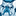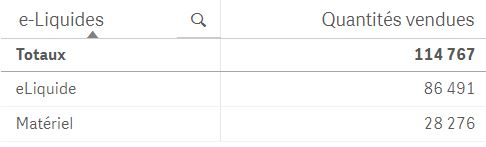Announcements
The way to achieve your own success is the willingness to help somebody else. Go for it!
cancel
Showing results for
Did you mean:Anonymous
Not applicable

## Only show certain value

Hi All,

I need help with an expression. I'm working on a dimension called "Level" and I just want to show where Level = 2 and exclude the rest of the Levels. I can do this by selection but I want to hard coded to just Level=2.

I tried with Only(Level)=2, it didn't works.  Any suggestions greatly appreciated!!!

Frank

1 Solution

Accepted SolutionsMVP

May be this as your calculated dimension

If(Level = 2, Level) and check 'Suppress When Value Is Null'

or use this as set analysis within your expression

{<Level = {2}>}

5 RepliesMVP

May be this as your calculated dimension

If(Level = 2, Level) and check 'Suppress When Value Is Null'

or use this as set analysis within your expression

{<Level = {2}>}Creator III

Hi

If you wanted to populate a list box with just Level 2 then you could use the expression function with something like:

=if(Level=2,Level)

This would then just give you a list box with that value.

If you wanted to code a chart/table to only display values filtered for Level 2 then use set analysis.

Example: SUM( {<Level= {2} >}Sales

Thanks

DanMaster II

You can do in two ways:

Option I:

Create a calculated dimension as If(Level=2,Level)

and make sure you put a checkmark against "Suppress when value is null"

Option I:

Dimension: Level

Expression: {<Level={2}>}Amount

Note: Please replace the field Amount with appropriate field in your table.

Hope this helps.Anonymous
Not applicable
Author

All,

All your responses works. Thank You!Creator III

Hello

I have a table and i want to show only two values "eLiqudie" and "Materiel" :When i use the function ONLY, it's work : =AGGR(ONLY({\$<[Cd. Univers (ref)]={'83','88'}>} [Lib. Univers (ref)]), [Lib. Univers (ref)])But when i use function ONLY in clause if results which obtain it's false :

=  if(\$(vELiquide)=10,

AGGR(ONLY({\$<[Cd. Univers (ref)]={'83'}>} [Lib. Rayon (ref)] ),[Lib. Rayon (ref)])

,

AGGR(ONLY({\$<[Cd. Univers (ref)]={'83','88'}>} [Lib. Univers (ref)]), [Lib. Univers (ref)])

)Community Browser